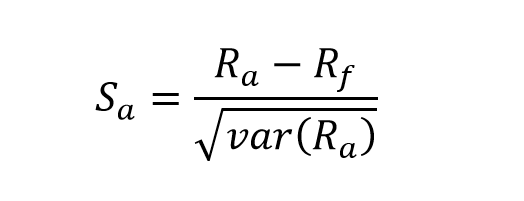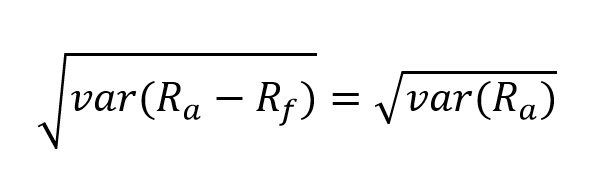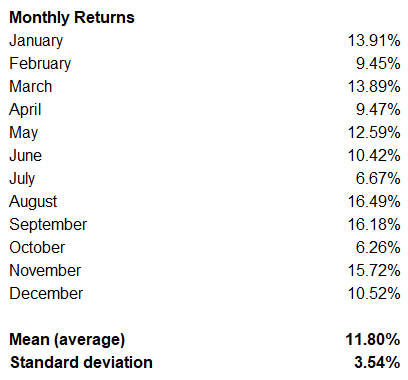## Sharpe Ratio and Risk-Adjusted Returns

In finance, one of the popular methods to adjust return rates of investments for risk is the Sharpe Ratio.

William F. Sharpe developed the ratio in 1966 and revised it in 1994 to arrive at the formula we use today. Originally he called it the ‘reward-to-variability’ ratio. Later on, finance professionals started referring to it as the Sharpe Ratio.

Investors use the Sharpe Ratio to understand how the return of investment compares to its risk. It’s the average return above the risk-free rate per unit of volatility or total risk. And volatility is a measure of the asset’s price fluctuations. A higher Sharpe Ratio means the investment generates a better risk-adjusted return.

## Understanding the Sharpe Ratio

As we discussed in our article on the Efficient Frontier and Optimal Portfolios, adding assets with low correlations to a portfolio can decrease the total risk without any loss in potential returns. When we increase the diversity within investments, this commonly results in a higher Sharpe Ratio. This holds right given the assumption that risk equals volatility, which may not be accurate for all investors.

The Sharpe Ratio shows us how much additional returns the investor will receive for the extra volatility endured holding a riskier asset. The ratio adjusts expected future returns or past performance for this additional investment risk.

We can apply the method to evaluate past performance (ex-post), or we can use expected future performance and the expected risk-free rate to estimate the Sharpe Ratio for the future (ex-ante).

When we apply the method to historical performance, the data has to cover a long enough period to accommodate all potential risks the investment strategy is taking. If we are looking at long-term assets, the past data may need to cover years. However, if our approach is one of high-frequency trading, then data for a few weeks might be sufficient.

The Sharpe Ratio can help in benchmarking investment opportunities. Whenever we compare two assets, the one with the higher ratio will either give better returns at the same risk level or the same profitability at a lower risk level.

### Limitations of the Sharpe Ratio

The main weakness of the method is that it uses the standard deviation of returns (or the square root of the variance) to denote total risk, assuming that investment returns follow a normal distribution. This means that the model associates equal risk to price movements in both directions. In reality, financial market returns are skewed away from the average, because of surprising shifts in price over time.

Portfolio managers, on the other hand, can manipulate their Sharpe Ratio by increasing the measuring interval, which results in a decreased volatility estimate. That way, they can significantly improve their risk-adjusted returns history. The annualized standard deviation of daily returns is usually higher than that of weekly returns, which in turn is higher than that of monthly returns.

## Sharpe Ratio Calculation

Initially, William F. Sharpe formulated the ratio asRa is the return of the evaluated asset. It can either be the average historical return over a specific period (ex-post) or the expected future return of the asset (ex-ante).

Rf is the risk-free rate. This is the return rate of assets with extremely low or virtually non-existent risk like government bonds. Usually, we would look into bonds of the same or close length as the investment under review.

We can also present the square root of the variance in the asset’s return as the standard deviation of the asset’s return – σa. The variance is widely popular as a measure of risk in Modern Portfolio Theory.

In 1994, Sharpe revised the method to have an applicable benchmark as the basis of comparison. The formula changed toRb is the return of the benchmark asset. Note that whenever Rb is the risk-free rate, thenWhen we calculate the ratio, we can use returns of any frequency (daily, weekly, monthly). This is because we can annualize the performance, given the assumption that it follows a normal distribution. And this is the main flaw of the Sharpe Ratio – not all investment returns follow such distributions.

## Analyzing the Sharpe Ratio

We can use the method to evaluate excess returns. It shows us whether these originated from a smart investment strategy, or there was too much risk involved. An asset can have a higher yield, but that’s only worth it if it doesn’t bring excessive amounts of additional risk.

Generally, we accept that the higher the Sharpe Ratio is, the better the risk-adjusted performance of the investment is.

An investment can have a negative ratio, showing the asset is underperforming against the benchmark. However, this gives us no real insights, as it means that either the risk-free rate is above the investment return, or the return is negative.

The typical Sharpe Ratio for the S&P500 index over ten years is around 0.5. An average diversified portfolio can go up to 0.75 to 1.00. We consider portfolios with ratios above 1.00 to about 2.00 to have an excellent performance. And almost all investment strategies that provide a Sharpe Ratio of more than 2.00 are within high-frequency trading.

The way to improve the Sharpe Ratio of any portfolio is diversifying to achieve an Optimal Portfolio that lies on the Efficient Frontier.

## Example

Let us look at a portfolio of investments that has the following monthly returns for the past year. From these, we can calculate our mean (average) return and the standard deviation of the returns.We now have the average return and the volatility of the profitability. Adding the risk-free rate of government bonds, we can calculate the Sharpe Ratio for the portfolio.A ratio of 1.5 suggests the outstanding performance of our portfolio. However, we might look in more detail and see if a monthly frequency is the most suitable for our calculations. As we discussed, increasing the measuring interval is a common way to improve our Sharpe Ratio artificially.

We are planning to add another investment to this portfolio and expect the return to drop to 10%. However, we also expect the volatility to drop to 2%, and the risk-free rate remains the same. Then, the calculation is as follows:While we have a lower return, the Sharpe Ratio has improved. The profitability of the portfolio will grow on a risk-adjusted basis with the additional investment.

We are looking in a simplified example to illustrate that, in its essence, the Sharpe Ratio tells us if the higher risk of some investments is indeed justified.

## Conclusion

When we evaluate investment opportunities, we must consider both reward and risk at the same time. The Sharpe Ratio helps us to determine the asset with the best return, while also taking risk into account. We can measure the performance of a portfolio against a risk-free asset, after adjusting for risk.

It represents the additional return an investor receives for each unit of increase in the risk they take, or how well the return rate compensates them for taking that risk.

Show your support by sharing the article with colleagues and friends via the social links on the left.

## And Get a FREE Benchmark Analysis Template## FCCA, FMVA

Hi! I am a finance professional with 10+ years of experience in audit, controlling, reporting, financial analysis and modeling. I am excited to delve deep into specifics of various industries, where I can identify the best solutions for clients I work with.

In my spare time, I am into skiing, hiking and running. I am also active on Instagram and YouTube, where I try different ways to express my creative side.